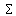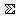# U+2140 DOUBLE-STRUCK N-ARY SUMMATION

U+2140 was added to Unicode in version 3.2 (2002). It belongs to the blockLetterlike Symbols in the Basic Multilingual Plane.

This character is a Math Symbol and is commonly used, that is, in no specific script.

The glyph is a Font composition of the glyphs. It has a Neutral East Asian Width. In bidirectional context it acts as Other Neutral and is mirrored. The glyph can, under circumstances, be confused with 12 other glyphs. In text U+2140 behaves as Alphabetic regarding line breaks. It has type Other for sentence and Other for word breaks. The Grapheme Cluster Break is Any.

In mathematics, summation (symbol: ) is the addition of a sequence of numbers; the result is their sum or total. If numbers are added sequentially from left to right, any intermediate result is a partial sum, prefix sum, or running total of the summation. The numbers to be summed (called addends, or sometimes summands) may be integers, rational numbers, real numbers, or complex numbers. Besides numbers, other types of values can be added as well: vectors, matrices, polynomials and, in general, elements of any additive group (or even monoid). For finite sequences of such elements, summation always produces a well-defined sum.

The summation of an infinite sequence of values is called a series. A value of such a series may often be defined, by means of a limit (although sometimes the value may be infinite, and often no value results at all). Another notion involving limits of finite sums is integration. The term summation has a special meaning related to extrapolation in the context of divergent series.

The summation of the sequence [1, 2, 4, 2] is an expression whose value is the sum of each of the members of the sequence. In the example, 1 + 2 + 4 + 2 = 9. Because addition is associative, the sum does not depend on how the additions are grouped, for instance (1 + 2) + (4 + 2) and 1 + ((2 + 4) + 2) both have the value 9; therefore, parentheses are usually omitted in repeated additions. Addition is also commutative, so permuting the terms of a finite sequence does not change its sum (for infinite summations this property may fail; see Absolute convergence for conditions under which it still holds).

There is no special notation for the summation of such explicit sequences, as the corresponding repeated addition expression will do. There is only a slight difficulty if the sequence has fewer than two elements: the summation of a sequence of one term involves no plus sign (it is indistinguishable from the term itself) and the summation of the empty sequence cannot even be written down (but one can write its value "0" in its place). If, however, the terms of the sequence are given by a regular pattern, possibly of variable length, then a summation operator may be useful or even essential. For the summation of the sequence of consecutive integers from 1 to 100 one could use an addition expression involving an ellipsis to indicate the missing terms: 1 + 2 + 3 + 4 + ... + 99 + 100. In this case the reader easily guesses the pattern; however, for more complicated patterns, one needs to be precise about the rule used to find successive terms, which can be achieved by using the summation operator "Σ". Using this sigma notation the above summation is written as:

The value of this summation is 5050. It can be found without performing 99 additions, since it can be shown (for instance by mathematical induction) that

for all natural numbers n (see Triangular number). More generally, formulae exist for many summations of terms following a regular pattern.

The term "indefinite summation" refers to the search for an inverse image of a given infinite sequence s of values for the forward difference operator, in other words for a sequence, called antidifference of s, whose finite differences are given by s. By contrast, summation as discussed in this article is called "definite summation".

When it is necessary to clarify that numbers are added with their signs, the term algebraic sum is used. For example, in electric circuit theory Kirchhoff's circuit laws consider the algebraic sum of currents in a network of conductors meeting at a point, assigning opposite signs to currents flowing in and out of the node.

## Representations

System Representation
8512
UTF-8 E2 85 80
UTF-16 21 40
UTF-32 00 00 21 40
URL-Quoted %E2%85%80
HTML-Escape &#x2140;
Wrong windows-1252 Mojibake â

## Complete Record

Property Value
Age (age) 3.2
Unicode Name (na) DOUBLE-STRUCK N-ARY SUMMATION
Unicode 1 Name (na1)
Block (blk) Letterlike_Symbols
General Category (gc) Math Symbol
Script (sc) Common
Bidirectional Category (bc) Other Neutral
Combining Class (ccc) Not Reordered
Decomposition Type (dt) Font
Decomposition Mapping (dm)Lowercase (Lower)
Simple Lowercase Mapping (slc)Lowercase Mapping (lc)Uppercase (Upper)
Simple Uppercase Mapping (suc)Uppercase Mapping (uc)Simple Titlecase Mapping (stc)Titlecase Mapping (tc)Case Folding (cf)ASCII Hex Digit (AHex)
Alphabetic (Alpha)
Bidi Control (Bidi_C)
Bidi Mirrored (Bidi_M)
Bidi Paired Bracket (bpb)Bidi Paired Bracket Type (bpt) None
Cased (Cased)
Composition Exclusion (CE)
Case Ignorable (CI)
Full Composition Exclusion (Comp_Ex)
Changes When Casefolded (CWCF)
Changes When Casemapped (CWCM)
Changes When NFKC Casefolded (CWKCF)
Changes When Lowercased (CWL)
Changes When Titlecased (CWT)
Changes When Uppercased (CWU)
Dash (Dash)
Deprecated (Dep)
Default Ignorable Code Point (DI)
Diacritic (Dia)
East Asian Width (ea) Neutral
Extender (Ext)
FC NFKC Closure (FC_NFKC)Grapheme Cluster Break (GCB) Any
Grapheme Base (Gr_Base)
Grapheme Extend (Gr_Ext)
Hex Digit (Hex)
Hangul Syllable Type (hst) Not Applicable
Hyphen (Hyphen)
ID Continue (IDC)
Ideographic (Ideo)
ID Start (IDS)
IDS Binary Operator (IDSB)
IDS Trinary Operator and (IDST)
InMC (InMC)
Indic Positional Category (InPC) NA
Indic Syllabic Category (InSC) Other
ISO 10646 Comment (isc)
Joining Group (jg) No_Joining_Group
Join Control (Join_C)
Jamo Short Name (JSN)
Joining Type (jt) Non Joining
Line Break (lb) Alphabetic
Logical Order Exception (LOE)
Math (Math)
Noncharacter Code Point (NChar)
NFC Quick Check (NFC_QC) Yes
NFD Quick Check (NFD_QC) Yes
NFKC Casefold (NFKC_CF)NFKC Quick Check (NFKC_QC) No
NFKD Quick Check (NFKD_QC) No
Numeric Type (nt) None
Numeric Value (nv) NaN
Other Alphabetic (OAlpha)
Other Default Ignorable Code Point (ODI)
Other Grapheme Extend (OGr_Ext)
Other ID Continue (OIDC)
Other ID Start (OIDS)
Other Lowercase (OLower)
Other Math (OMath)
Other Uppercase (OUpper)
Pattern Syntax (Pat_Syn)
Pattern White Space (Pat_WS)
Quotation Mark (QMark)
Sentence Break (SB) Other
Simple Case Folding (scf)Script Extension (scx) Common
Soft Dotted (SD)
STerm (STerm)
Terminal Punctuation (Term)
Unified Ideograph (UIdeo)
Variation Selector (VS)
Word Break (WB) Other
White Space (WSpace)
XID Continue (XIDC)
XID Start (XIDS)
Expands On NFC (XO_NFC)
Expands On NFD (XO_NFD)
Expands On NFKC (XO_NFKC)
Expands On NFKD (XO_NFKD)